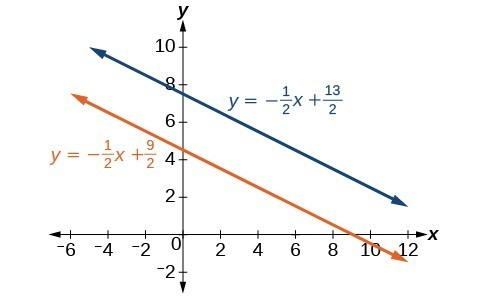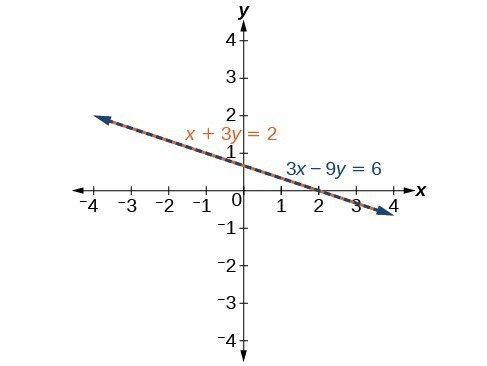Identifying and Expressing Solutions to Systems of Equations

Now that we have several methods for solving systems of equations, we can use the methods to identify inconsistent systems. Recall that an inconsistent system consists of parallel lines that have the same slope but different $y$ -intercepts. They will never intersect. When searching for a solution to an inconsistent system, we will come up with a false statement, such as $12=0$.

Example 8: Solving an Inconsistent System of Equations

Solve the following system of equations.

$\begin{array}{l}\text{ }x=9 - 2y\hfill \\ x+2y=13\hfill \end{array}$

Solution

We can approach this problem in two ways. Because one equation is already solved for $x$, the most obvious step is to use substitution.

$\begin{array}{l}x+2y=13\hfill \\ \left(9 - 2y\right)+2y=13\hfill \\ 9+0y=13\hfill \\ 9=13\hfill \end{array}$

Clearly, this statement is a contradiction because $9\ne 13$. Therefore, the system has no solution.

The second approach would be to first manipulate the equations so that they are both in slope-intercept form. We manipulate the first equation as follows.

$\begin{array}{l}\text{ }x=9 - 2y\hfill \\ 2y=-x+9\hfill \\ \text{ }y=-\frac{1}{2}x+\frac{9}{2}\hfill \end{array}$

We then convert the second equation expressed to slope-intercept form.

$\begin{array}{l}x+2y=13\hfill \\ \text{ }2y=-x+13\hfill \\ \text{ }y=-\frac{1}{2}x+\frac{13}{2}\hfill \end{array}$

Comparing the equations, we see that they have the same slope but different y-intercepts. Therefore, the lines are parallel and do not intersect.

$\begin{array}{l}\begin{array}{l}\\ y=-\frac{1}{2}x+\frac{9}{2}\end{array}\hfill \\ y=-\frac{1}{2}x+\frac{13}{2}\hfill \end{array}$

Analysis of the Solution

Writing the equations in slope-intercept form confirms that the system is inconsistent because all lines will intersect eventually unless they are parallel. Parallel lines will never intersect; thus, the two lines have no points in common. The graphs of the equations in this example are shown in Figure 8.Figure 8

Try It 6

Solve the following system of equations in two variables.

$\begin{array}{l}2y - 2x=2\\ 2y - 2x=6\end{array}$

Solution

Expressing the Solution of a System of Dependent Equations Containing Two Variables

Recall that a dependent system of equations in two variables is a system in which the two equations represent the same line. Dependent systems have an infinite number of solutions because all of the points on one line are also on the other line. After using substitution or addition, the resulting equation will be an identity, such as $0=0$.

Example 9: Finding a Solution to a Dependent System of Linear Equations

Find a solution to the system of equations using the addition method.

$\begin{array}{c}x+3y=2\\ 3x+9y=6\end{array}$

Solution

With the addition method, we want to eliminate one of the variables by adding the equations. In this case, let’s focus on eliminating $x$. If we multiply both sides of the first equation by $-3$, then we will be able to eliminate the $x$ -variable.

$\begin{array}{l}\text{ }x+3y=2\hfill \\ \left(-3\right)\left(x+3y\right)=\left(-3\right)\left(2\right)\hfill \\ \text{ }-3x - 9y=-6\hfill \end{array}$

$\begin{array} \hfill−3x−9y=−6 \\ \hfill+3x+9y=6 \\ \hfill \text{_____________} \\ \hfill 0=0 \end{array}$

We can see that there will be an infinite number of solutions that satisfy both equations.

Analysis of the Solution

If we rewrote both equations in the slope-intercept form, we might know what the solution would look like before adding. Let’s look at what happens when we convert the system to slope-intercept form.

$\begin{array}{l}\text{ }x+3y=2\hfill \\ \text{ }3y=-x+2\hfill \\ \text{ }y=-\frac{1}{3}x+\frac{2}{3}\hfill \\ 3x+9y=6\hfill \\ \text{ }9y=-3x+6\hfill \\ \text{ }y=-\frac{3}{9}x+\frac{6}{9}\hfill \\ \text{ }y=-\frac{1}{3}x+\frac{2}{3}\hfill \end{array}$

See Figure 9. Notice the results are the same. The general solution to the system is $\left(x, -\frac{1}{3}x+\frac{2}{3}\right)$.Figure 9

Try It 7

Solve the following system of equations in two variables.

$\begin{array}{l}\begin{array}{l}\\ \text{ }\text{}\text{}y - 2x=5\end{array}\hfill \\ -3y+6x=-15\hfill \end{array}$